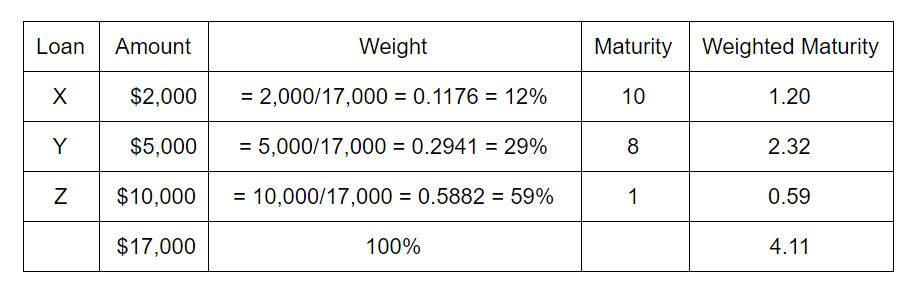# Weighted Average Maturity (WAM)

Updated November 4, 2020

## What Is Weighted Average Maturity?

Weighted average maturity or WAM is the weighted average amount of time until the securities in a portfolio mature.

The higher the WAM, the longer it takes for all of the holdings in the portfolio to mature. WAM is used to manage debt portfolios and to evaluate the profitability of the portfolio managers.

## How Does Weighted Average Maturity Work?

The WAM can be useful to investors in bond portfolios or in securities that are backed by debt securities, such as mortgage-backed

## Weighted Average Maturity Calculation with Example

The WAM can be calculated by determining the weight of each maturity in the average, multiplying that weight by the security’s maturity, and summing the weighted maturities. The weight is the proportion of total portfolio value that each security represents.

For example, assume you want to find the WAM of three loans, Loan X is for \$2,000 that matures in 10 years, Loan Y is for \$5,000 and matures in 8 years, and Loan Z is for \$10,000 and matures in 1 year:• Determine the weight of each loan by finding its percentage of the total amount, in this case, \$17,000.
• Now, multiply the weight of each loan by the number of years left until the loan’s maturity.
• Add these weighted maturities to determine the WAM.

The portfolio of loans will mature in 4.11 years, according to its weighted average.

All of our content is verified for accuracy by Rachel Siegel, CFA and our team of certified financial experts. We pride ourselves on quality, research, and transparency, and we value your feedback. Below you'll find answers to some of the most common reader questions about Weighted Average Maturity (WAM).
##### Be the first to ask a question

Rachel Siegel, CFA - 141Rachel Siegel, CFACFA Charterholder

Chartered Financial Analyst

Rachel Siegel, CFA is one of the nation's leading experts at ensuring the accuracy of financial and economic text.  Her prestigious background includes over 10 years of experience in creating professional financial certification exams and another 20 years of college-level teaching.